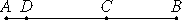# Proposition 27

If an even number is subtracted from an odd number, then the remainder is odd.

Let the even number BC be subtracted from the odd number AB.

I say that the remainder CA is odd.VII.Def.7

Subtract the unit AD, therefore DB is even.

But BC is also even, therefore the remainder CD is even. Therefore CA is odd.

Therefore, if an even number is subtracted from an odd number, then the remainder is odd.

Q.E.D.

## Guide

This is the last of four propositions that examine the result of subtracting even and odd numbers from each other.